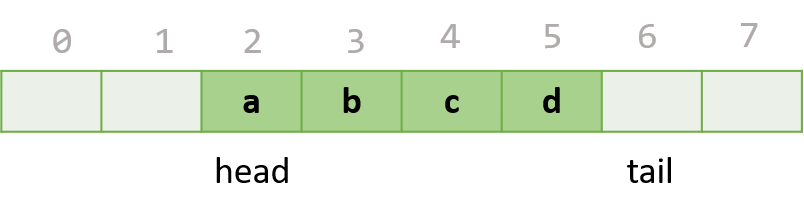## Data structures, a queue using arrays in C++

Last time we took a look at a simple linear queue with a list using Python, this implementation was super simple and basically we have to care nothing about the size or capacity of the queue. This is fantastic and I am pretty sure most of the time you won’t have to take care of the capacity of the queue.

Using a list for a queue is fantastic when we don’t know the final size or maximum size of our queue, but sometimes we know beforehand what is the maximum capacity available for processing, for this cases a much more efficient way would be using an array as the internal container facility for our queue, remember, in languages like C++ and Go you cannot resize arrays dynamically. The usage is simple, we create a queue with a max capacity of 10 and when we try to add the 11 element it will report the error because our queue is full.A quick implementation would require two indices to store the current positions of head and tail (remember, in a queue we add elements to the tail and pop elements from the head) and probably we will use a simple boolean check to see if we passed the capacity. This implementation has a flaw though as I will exemplify with the following steps:

1. We create a queue with a capacity of 2
4. We pop one element
5. We try to add another element

In the fifth step our queue will throw an exception because we had surpassed its capacity, that is not true because we already dequeued one element so we basically has space to fit another element in the queue, can you see the problem?

A simple way to solve this is using a circular index for both tail and head and we can calculate the next position using a simple modular operation (next = (current + 1) % size), well it is a reminder operation not a modulus but I already ranted about that in the past. This moving indices is called a chasing pointer where the head index is chasing the tail index, every time we add an element we move the tail and every time we dequeue we have to move the head.

This implementation still has a very small but not so nice issue, we know the queue is empty if the tail and top points to the same index but this case will happen as well when the queue is full. There are a few ways to solve this issue but the one I liked the most (after reading Cormen and a very good old book about data structures, Practical data structures in C++).

For the implementation I used C++, which think it illustrates pretty well this specific case, I hope it helps you to see it more clearly.

#pragma once
#include <array>
#include <exception>

template<typename T, size_t N>
class FixedSizeQueue {
std::array<T, N> elements{};
bool isFull{false};
size_t tail{0};
public:
FixedSizeQueue() = default;
~FixedSizeQueue() = default;

[[nodiscard]] bool isEmpty() const noexcept {
return head == tail && !isFull;
}

T dequeue() {
if (isEmpty()) throw std::out_of_range("Queue is empty");
if (head == tail) isFull = false; // NOTE: We reached the tail, we are empty

return item;
}

T peek() const {
if (isEmpty()) throw std::out_of_range("Queue is empty");
}

void enqueue(const T& element) {
if (isFull) throw std::out_of_range("Queue is full");
elements[tail] = element;
tail = (tail + 1)%N;
if (tail == head) isFull = true; // NOTE: We reached the head, we are full
}

[[nodiscard]] bool IsFull() const noexcept {
return isFull;
}
};


And the unit tests (using Catch2) for our implementation is a little verbose but hey, it is C++ after all!

#define CATCH_CONFIG_MAIN
#include <catch2/catch.hpp>
#include "CircularQueue.h"

TEST_CASE("It is empty", "[circular queue]") {
CircularQueue<int, 10> queue;
CHECK(queue.isEmpty());
}

TEST_CASE("Normal enqueue-dequeue", "[circular queue]") {
CircularQueue<int, 10> queue;
queue.enqueue(1);
queue.enqueue(2);
CHECK_FALSE(queue.isEmpty());
REQUIRE(queue.dequeue() == 1);
CHECK_FALSE(queue.isEmpty());
REQUIRE(queue.dequeue() == 2);
CHECK(queue.isEmpty());
}

TEST_CASE("Peek doesn't dequeue", "[circular queue]") {
CircularQueue<int, 10> queue;
queue.enqueue(1);
REQUIRE(queue.peek() == 1);
CHECK_FALSE(queue.isEmpty());
}

TEST_CASE("Dequeue and peek throw if empty", "[circular queue]") {
CircularQueue<int, 10> queue;
REQUIRE_THROWS_WITH(queue.dequeue(), "Queue is empty");
REQUIRE_THROWS_WITH(queue.peek(), "Queue is empty");
}

TEST_CASE("When queue limit is reached, we discard top and move", "[circular queue]") {
CircularQueue<int, 2> queue;
queue.enqueue(1);
queue.enqueue(2);
REQUIRE_THROWS_WITH(queue.enqueue(3), "Queue is full");
}


In the same way as with Python, the standard library in C++ has a really good and powerful implementation for queues using an underline container, as usual, use any of those instead of this sample code!

PD: Yes, I know we could use slices in Go or std::vector in C++, but that is not the point of this post.